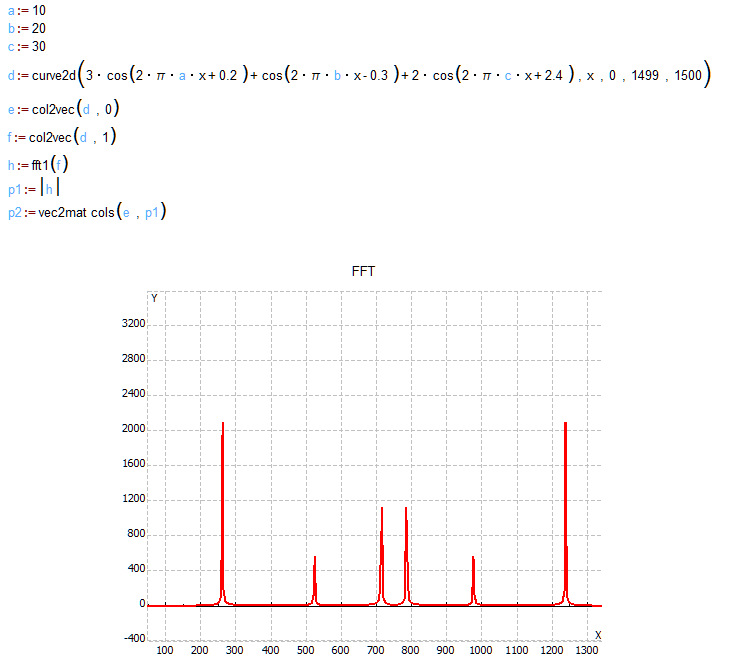# FFT – Fast Fourier Transform

Fast Fourier Transform – FFT is widely used for many applications in engineering, science, and mathematics. FFT is an algorithm that is used to calculate the frequency components of the input signal. FFT computes discrete Fourier transform of an input sequence. It is very important to use an efficient algorithm for rapid calculations, and decrease the algorithmic complexity from an original O (n2) to an effective O (nlogn).MatDeck’s function fft1() rapidly calculates the DFT of the input sequence, with speed comparable to other leading mathematical software achieving 0.5 sec for the sequence length equal to one million samples . This speed is achieved by using the “Build and Run Exe” option to execute the fft1 () function. Function, ffti1 (), is used to compute the inverse discrete Fourier transform, and to move from frequency domain to time domain. Function, ffti1 (), is built with a very efficient algorithm as well. All MatDeck functions that rely on fft1 () or ffti1() are extremely efficient in the sense of algorithms.